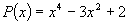I. INTRODUCTORY PROBLEMS

1. (i) What is the condition for the quadratic polynomial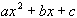to have (a) no real root, (b) two real zeros ?

(ii) How many real zeros have the polynomials:

(a)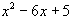(b)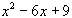(c)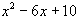(d)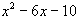where they exist, find the root(s).

(iii) Find the real zeros of the following polynomials:

(a)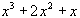(b)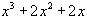(c)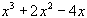2. (i) Show that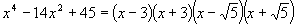, and hence state the number of real zeros of the polynomial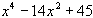.

(ii) Determine the real zeros of the polynomials

(a)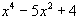(b)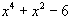(c)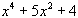3. Determine the zeros of the polynomials P(x) and hence sketch the curve y = P(x)

(a)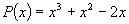(b)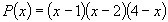(c)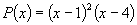(d)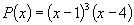(e)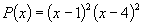(f)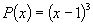4. Sketch the following curves:

(a)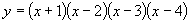(b)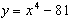hence determine the set of values of x for which the polynomials

(i)< 0 (ii)> 0

5. Find the real zeros of the following polynomials P(x) and hence sketch the curve:

(a)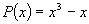(b)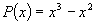(c)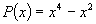(d)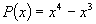(e)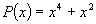(f)